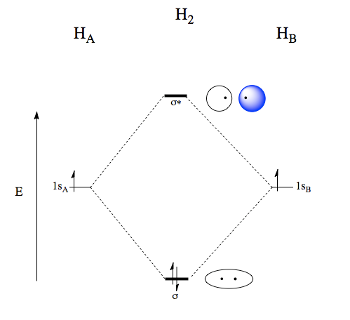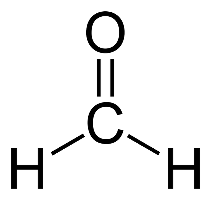# Bond Order Formula, Calculation, & Examples

Jayashree Raman, Nancy Devino
• Author
Jayashree Raman

Jayashree has taught high school chemistry for over thirty years. She has a bachelor's degree in chemistry and master's degree in English from Madurai University. She has a teaching certification, Bachelor of Education, from University of Delhi.

• Instructor
Nancy Devino
Explore bond orders. Learn the definition of bond order and see the formula to calculate bond order. Understand why the calculation of bond order is important. Updated: 11/03/2021

Show

## What is Bond Order?

A measurement of the number of electron pairs that form bonds between two atoms gives the bond order. Linus Pauling introduced the term bond order.

The definition of bond order is "the value of one-half of the difference between the number of bonding electrons and antibonding electrons in a molecule."

When considering Lewis structures, bond order is the number of bonds between the two given atoms per domain.

Or, simply put, the bond order of two atoms having a single bond between them is one. Again, if two atoms have a double bond between them, the bond order is two. Similarly, the bond order is three if two atoms have a triple bond between them.An error occurred trying to load this video.

Try refreshing the page, or contact customer support.

Coming up next: Equilibrium Constant & Cell Potential

### You're on a roll. Keep up the good work!

Replay
Your next lesson will play in 10 seconds
• 0:00 Single, Double, or…
• 0:46 Calculating Bond Order
• 2:03 Non-Integral Bond Order
• 3:33 Lesson Summary
Save Save

Want to watch this again later?

Timeline
Autoplay
Autoplay
Speed Speed

## Bond Order Formula

In the molecular orbital theory, two atomic orbitals of valence electrons of similar energy and orientation form a bond. Molecular orbital arises from two atomic orbitals, which have to be conserved. So, there is a bonding orbital and an antibonding orbital.

The bonding orbital is a three-dimensional volume of space where the electrons move, experiencing the attractive force of the nuclei of both atoms involved in bonding. The electron density is maximum in this region.

The antibonding orbital is the space between the nuclei where the probability of finding electrons is zero. This space is the nodal plane. The electron density is higher at places away from the nuclei.

The antibonding orbitals have an asterisk to distinguish them from bonding orbitals.

For determining the bonding and antibonding orbitals, molecular orbital diagrams are necessary.

• Draw the atomic orbitals on the sides.
• The molecular orbitals are in the center brought together by joining the corresponding atomic orbitals.
• The bonding orbitals have lower energy and more stability than the atomic orbitals. So they are at a lower level than the atomic orbitals.
• The antibonding orbitals have more energy than their parent atomic orbitals. So they are above the atomic orbitals. They have an asterisk.
• The orbitals fill up according to the Aufbau principle.

The following illustrates how to find the bond order of molecules.

Example

Molecule of hydrogen.

There are two atoms, and each atom has one 1s electron.

The two atomic orbitals approach each other as they are similar in energy and orientation.

They form a molecular bonding orbital.

There are no electrons for antibonding orbitals, so it is empty.For multi-electron atoms, draw the diagram only for the valence electrons.

The formula for determining the bond order considers the valence electrons in the bonding and antibonding orbitals.

The formula for bond order calculation is half the difference between the number of electrons in the bonding and antibonding orbitals.

Mathematically the formula is

{eq}(Number of bonding electrons - number of antibonding electrons)\div 2 {/eq}

Using this formula, we can calculate the bond order in different molecules.

In the case of hydrogen:

bonding electrons = 2

anti-bonding electrons = 0

Bond Order = (2-0) /2 =1

There is a single bond between the atoms.

## Bond Order Calculation

Atoms form bonds using their valence electrons. All electrons do not have the same energy. Energy differs among orbitals within an energy level. An atom, while bonding, may try to equalize the energy of two or more of its electrons in different orbitals by mixing them or by hybridizing them. For example, carbon atom shows hybridization. If one s and one p orbitals undergo hybridization, it is sp hybridization. Mixing of one s with two p, and one s with three p orbitals gives {eq}sp^{2} {/eq} and {eq}sp^{3} {/eq} hybridization. After hybridization, the orbitals have equal energy and are degenerate.

Resonance occurs when a molecule has two or more equivalent contributing structures. The electrons delocalize and spread over atoms. The resonance structures help explain properties that do not conform to specific Lewis structures. Also, the resonance hybrid structure gives more stability, and the properties are intermediate to the contributing forms.

With these in mind, it is not surprising that bond order values sometimes come out as fractions, non-integrals. Benzene, ozone, and ions like carbonate and sulfate show resonance.

Take the following steps to find the bond order between two specific atoms in a polyatomic molecule or ions.

1. Draw the Lewis structure of the compound or ion.
2. Count the total number of bonds between the atoms concerned.
3. Determine how many such groups of bonds there are.
4. Divide the total number of bonds by the number of groups or domains of bonds to get the bond order.

## Bond Order Examples

Here are some examples of how to find bond order in molecules.

Example 1

Nitrogen molecule

The Lewis structure shows six electrons shared between the two atoms. It is a triple bond.There are three bonds over one group of atoms. Applying the formula,

{eq}\left ( Number of bonds\div \div Number of groups \right ) {/eq},

{eq}3\ast 1= 3 {/eq}

The bond order is three.

Example 2

Formaldehyde

Its formula is HCHO.To determine the bond order between the carbon and oxygen atom, notice it is a double bond. The group or domain is just one. So the bond order is

{eq}2\div 1= 2 {/eq}

The bond order is two.

Example 3

Benzene.

To unlock this lesson you must be a Study.com Member.

#### What does bond order mean?

Bond order is the number of bonds that two atoms form in a molecule. Bond order is just the number of bonds between two atoms when they combine. Bond order values may be whole numbers or non- integrals.

#### What does bond order tell you?

Bond order tells us about the nature of bonds present between the atoms specified. For example, if the bond order between two atoms of X is 1, it means there is a single bond between the two atoms of X.

Similarly, a bond order of two denotes the presence of a double bond.

#### How do you find the bond order?

The first step is to draw the molecular orbital diagram, filling the orbitals in increasing order of energy. The inner core electrons are already in paired form.

Add up the total number of bonding and antibonding electrons. Substitute these values in the formula

Bond Order = (Number of bonding electrons - number of antibonding electrons) /2.

The answer gives the bond order.

### Register to view this lesson

Are you a student or a teacher?